Anúncio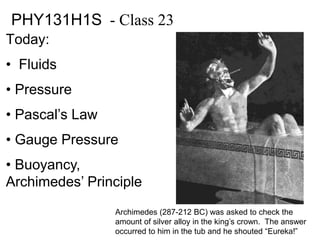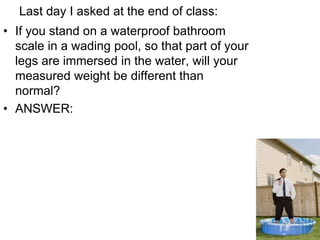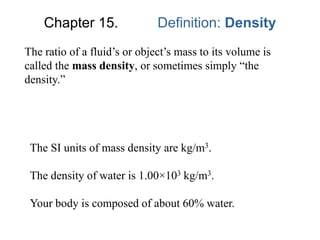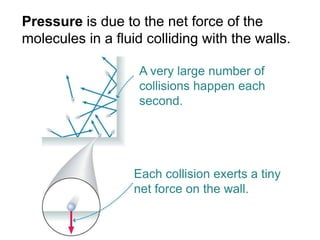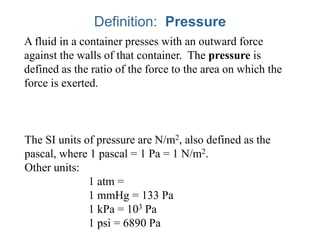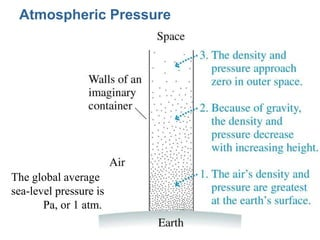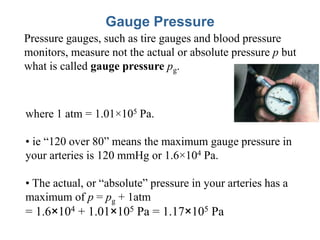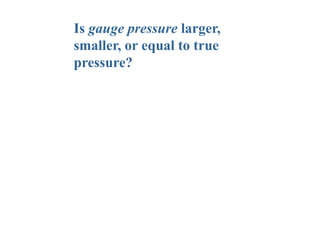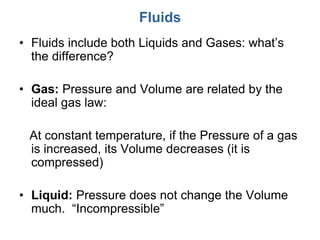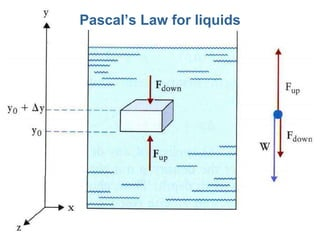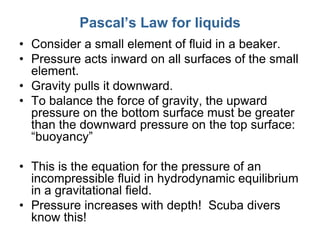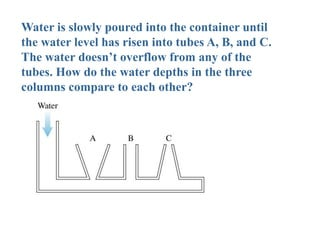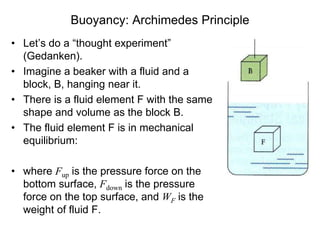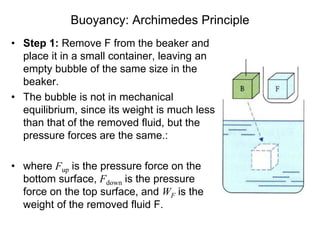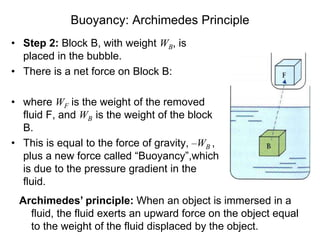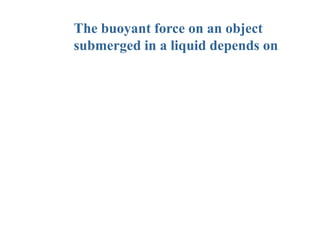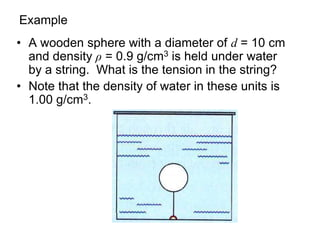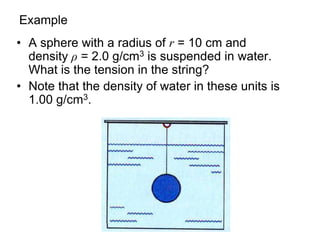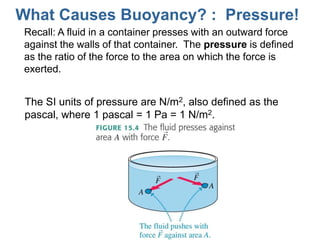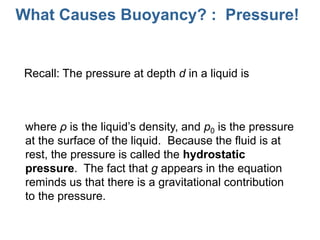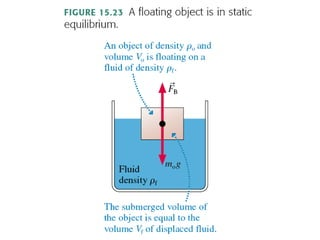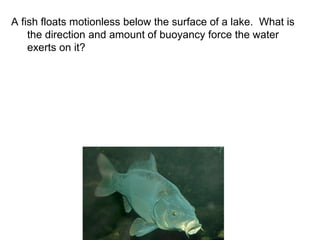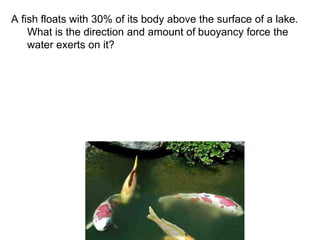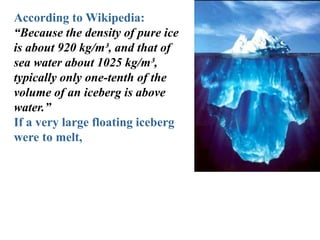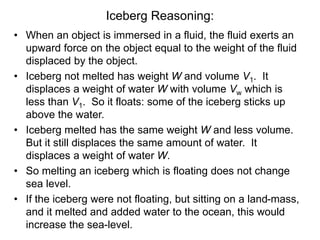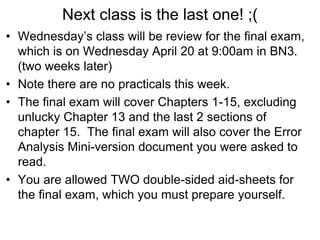1 de 27
Anúncio

### Lec23skel

1. PHY131H1S - Class 23 Today: • Fluids • Pressure • Pascal’s Law • Gauge Pressure • Buoyancy, Archimedes’ Principle Archimedes (287-212 BC) was asked to check the amount of silver alloy in the king’s crown. The answer occurred to him in the tub and he shouted “Eureka!”
2. A little pre-class reading quiz…
3. Last day I asked at the end of class: • If you stand on a waterproof bathroom scale in a wading pool, so that part of your legs are immersed in the water, will your measured weight be different than normal? • ANSWER:
4. Chapter 15. Definition: Density The ratio of a fluid’s or object’s mass to its volume is called the mass density, or sometimes simply “the density.” The SI units of mass density are kg/m3. The density of water is 1.00×103 kg/m3. Your body is composed of about 60% water.
5. Pressure is due to the net force of the molecules in a fluid colliding with the walls. A very large number of collisions happen each second. Each collision exerts a tiny net force on the wall.
6. Definition: Pressure A fluid in a container presses with an outward force against the walls of that container. The pressure is defined as the ratio of the force to the area on which the force is exerted. The SI units of pressure are N/m2, also defined as the pascal, where 1 pascal = 1 Pa = 1 N/m2. Other units: 1 atm = 1 mmHg = 133 Pa 1 kPa = 103 Pa 1 psi = 6890 Pa
7. Atmospheric Pressure The global average sea-level pressure is Pa, or 1 atm.
8. Gauge Pressure Pressure gauges, such as tire gauges and blood pressure monitors, measure not the actual or absolute pressure p but what is called gauge pressure pg. where 1 atm = 1.01×105 Pa. • ie “120 over 80” means the maximum gauge pressure in your arteries is 120 mmHg or 1.6×104 Pa. • The actual, or “absolute” pressure in your arteries has a maximum of p = pg + 1atm = 1.6×104 + 1.01×105 Pa = 1.17×105 Pa
9. Is gauge pressure larger, smaller, or equal to true pressure?
10. Fluids • Fluids include both Liquids and Gases: what’s the difference? • Gas: Pressure and Volume are related by the ideal gas law: At constant temperature, if the Pressure of a gas is increased, its Volume decreases (it is compressed) • Liquid: Pressure does not change the Volume much. “Incompressible”
11. Pascal’s Law for liquids
12. Pascal’s Law for liquids • Consider a small element of fluid in a beaker. • Pressure acts inward on all surfaces of the small element. • Gravity pulls it downward. • To balance the force of gravity, the upward pressure on the bottom surface must be greater than the downward pressure on the top surface: “buoyancy” • This is the equation for the pressure of an incompressible fluid in hydrodynamic equilibrium in a gravitational field. • Pressure increases with depth! Scuba divers know this!
13. Water is slowly poured into the container until the water level has risen into tubes A, B, and C. The water doesn’t overflow from any of the tubes. How do the water depths in the three columns compare to each other?
14. Buoyancy: Archimedes Principle • Let’s do a “thought experiment” (Gedanken). • Imagine a beaker with a fluid and a block, B, hanging near it. • There is a fluid element F with the same shape and volume as the block B. • The fluid element F is in mechanical equilibrium: • where Fup is the pressure force on the bottom surface, Fdown is the pressure force on the top surface, and WF is the weight of fluid F.
15. Buoyancy: Archimedes Principle • Step 1: Remove F from the beaker and place it in a small container, leaving an empty bubble of the same size in the beaker. • The bubble is not in mechanical equilibrium, since its weight is much less than that of the removed fluid, but the pressure forces are the same.: • where Fup is the pressure force on the bottom surface, Fdown is the pressure force on the top surface, and WF is the weight of the removed fluid F.
16. Buoyancy: Archimedes Principle • Step 2: Block B, with weight WB, is placed in the bubble. • There is a net force on Block B: • where WF is the weight of the removed fluid F, and WB is the weight of the block B. • This is equal to the force of gravity, –WB , plus a new force called “Buoyancy”,which is due to the pressure gradient in the fluid. Archimedes’ principle: When an object is immersed in a fluid, the fluid exerts an upward force on the object equal to the weight of the fluid displaced by the object.
17. The buoyant force on an object submerged in a liquid depends on
18. Example • A wooden sphere with a diameter of d = 10 cm and density ρ = 0.9 g/cm3 is held under water by a string. What is the tension in the string? • Note that the density of water in these units is 1.00 g/cm3.
19. Example • A sphere with a radius of r = 10 cm and density ρ = 2.0 g/cm3 is suspended in water. What is the tension in the string? • Note that the density of water in these units is 1.00 g/cm3.
20. What Causes Buoyancy? : Pressure! Recall: A fluid in a container presses with an outward force against the walls of that container. The pressure is defined as the ratio of the force to the area on which the force is exerted. The SI units of pressure are N/m2, also defined as the pascal, where 1 pascal = 1 Pa = 1 N/m2.
21. What Causes Buoyancy? : Pressure! Recall: The pressure at depth d in a liquid is where ρ is the liquid’s density, and p0 is the pressure at the surface of the liquid. Because the fluid is at rest, the pressure is called the hydrostatic pressure. The fact that g appears in the equation reminds us that there is a gravitational contribution to the pressure.
22. A fish floats motionless below the surface of a lake. What is the direction and amount of buoyancy force the water exerts on it?
23. A fish floats with 30% of its body above the surface of a lake. What is the direction and amount of buoyancy force the water exerts on it?
24. According to Wikipedia: “Because the density of pure ice is about 920 kg/m³, and that of sea water about 1025 kg/m³, typically only one-tenth of the volume of an iceberg is above water.” If a very large floating iceberg were to melt,
25. Iceberg Reasoning: • When an object is immersed in a fluid, the fluid exerts an upward force on the object equal to the weight of the fluid displaced by the object. • Iceberg not melted has weight W and volume V1. It displaces a weight of water W with volume Vw which is less than V1. So it floats: some of the iceberg sticks up above the water. • Iceberg melted has the same weight W and less volume. But it still displaces the same amount of water. It displaces a weight of water W. • So melting an iceberg which is floating does not change sea level. • If the iceberg were not floating, but sitting on a land-mass, and it melted and added water to the ocean, this would increase the sea-level.
26. Next class is the last one! ;( • Wednesday’s class will be review for the final exam, which is on Wednesday April 20 at 9:00am in BN3. (two weeks later) • Note there are no practicals this week. • The final exam will cover Chapters 1-15, excluding unlucky Chapter 13 and the last 2 sections of chapter 15. The final exam will also cover the Error Analysis Mini-version document you were asked to read. • You are allowed TWO double-sided aid-sheets for the final exam, which you must prepare yourself.
Anúncio